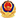##### Cost quick calculation

In case of any discrepancy between the following calculation results and those of the case handling system of the filing Department of this Council, the calculation of the filing department shall prevail.

•• Amount in dispute
• Acceptance fee
• Treatment fee
• Total
Standard of case acceptance fee
• Amount in dispute (RMB)
• Charging standard
• Arbitration case acceptance fee (RMB)
• Less than 1000 yuan
• 100yuan
• 100yuan
• 1000 yuan to 50000 yuan
• 5%
• 100 yuan + 5% of the disputed amount above 1000 yuan
• 50000 to 100000 yuan
• 4%
• 2550 yuan + 4% of the dispute amount above 50000 yuan
• 100000 to 200000 yuan
• 3%
• 4550 yuan + 3% of the dispute amount above 100000 yuan
• 200000 to 500000 yuan
• 2%
• 2550 yuan + 4% of the dispute amount above 50000 yuan
• RMB 500000 to RMB 1 million
• 1%
• 13550 yuan + 1% of the disputed amount above 500000 yuan
• More than 1 million yuan
• 0.5%
• 18550 yuan + 0.5% of the disputed amount above 1 million yuan
Standard of case handling fee
• Amount in dispute (RMB)
• Charging standard
• Case acceptance fee (RMB)
• Less than 50000 yuan
• The minimum is not less than 1000 yuan
• 50000 to 200000 yuan
• 0.9%
• RMB 1000 + 0.9% of the disputed amount above RMB 50000
• 200000 to 500000 yuan
• 0.7%
• 2350 yuan + 0.7% of the dispute amount above 200000 yuan
• RMB 500000 to RMB 1 million
• 3%
• 4450 yuan + 0.5% of the dispute amount above 500000 yuan
• 1 million to 3 million yuan
• 0.2%
• 6950 yuan + 0.2% of the disputed amount above 1 million yuan
• 3 million to 6 million yuan
• 0.18%
• 13550 yuan + 1% of the disputed amount above 500000 yuan
• 6 million to 10 million yuan
• 0.15%
• 16350 yuan + 0.15% of the disputed amount above 6 million yuan
• More than 1 million yuan
• 0.5%
• 10950 yuan + 0.18% of the disputed amount above 3 million yuan
• 10 million to 20 million yuan
• 0.12%
• 22350 yuan + 0.12% of the disputed amount above 10 million yuan
• 20 million to 40 million yuan
• 0.08%
• 34350 yuan + 0.08% of the disputed amount above 20 million yuan
• More than 40 million yuan
• 0.05%
• 50350 yuan + 0.05% of the disputed amount above 40 million yuan

Note: the above expenses are calculated by the subject amount

Copyright 石家庄仲裁委员会 Website ID：13010202001539 冀ICP备10005293号-1冀公网安备 13010202001539号